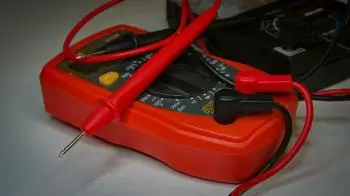# State Joule’s Law of Heating. What Is Joule's Effect?

The Joule effect, also called Joule's law, is the thermal manifestation of electrical resistance. It states that the heat generated due to the current that flows along a conductor is proportional to the electrical resistance, the amperage, and the time.Joule's law also states that the internal energy of an ideal gas is solely a function of its temperature.

If electricity circulates in an electrical conductor, a part of the kinetic energy of the electrons is transformed into heat, raising the temperature of the conductor.

The heat is generated due to the collision that electrons experience with the molecules of the conductive material through which they circulate.

Joule's law is named after the English physicist James Prescott Joule.

Joule's first law is also sometimes known as the Joule-Lenz law, because it was later found independent by Heinrich Lenz.

Joule also defined the Joule-Thomson effect of an ideal gas.

## What Is the Definition of the Joule Effect?

The Joule's law of heating states that the amount of heat energy produced by an electric current is directly proportional to the square of the intensity of the current when it circulates through the conductor and to the resistance that this conductor opposes to the passage of current.

The amount of heat produced in an electrical circuit is proportional to the time, the resistance of the wire, and the amperage.

## What Is the Formula for the Joule Effect?

From the previous definition, the formula of Joule's law can be expressed as follows:

Q = I 2  R t

where:

• Q = Heat produced in Joules (J) produced by the current.

• I = Intensity of the circulating current expressed in amperes (A)

• R = Electrical resistance of the conductor expressed in ohms.

• t = Time, in seconds (s)

## Examples of Joule's Law

• Filament bulbs: The filament is a resistance that heats up until it becomes incandescent with the current passage. As can be seen, the amount of heat emitted by the bulb is proportional to the filament's resistance.

• Household appliances that generate heat: Many household appliances rely on the Joule effect to function: electric ovens, toasters, electric heaters. In all these cases, it is intended to generate thermal energy with the passage of electricity through its conductors. This heat they give off is due to the Joule effect.

• The efficiency of electric motors depends on Joule's law.

## What Is the Downside of the Joule Effect?

The main drawback of the joule effect is the loss of energy that is transformed into heat and the overheating of electrical systems.

In the vast majority of applications, the Joule effect is undesirable. For this reason, some electrical and electronic devices need heat sinks to avoid excessive heating of the different components and devices.

Part of the electrical power supplied is converted into thermal energy, which is dissipated as heat. Therefore, the heat produced is wasted energy and, consequently, a decrease in its efficiency.

## Why Is Electricity Transmitted over High Voltage Lines?

Overhead power lines transfer electrical energy from electricity producers to consumers. However, these power lines have a non-zero resistance and are subject to the Joule effect, causing transmission losses.

There are two solutions to minimize transmission losses:

• First, reduce the resistance of the conductors. Line resistance is minimized through the use of copper conductors.

• Increase the voltage to lower the current intensity according to Ohm's law. Lowering the amperage decreases the number of electrons that circulate through the conductor and, therefore, the collisions against the conductor are reduced.

Author:

Published: September 14, 2021
Last review: September 14, 2021